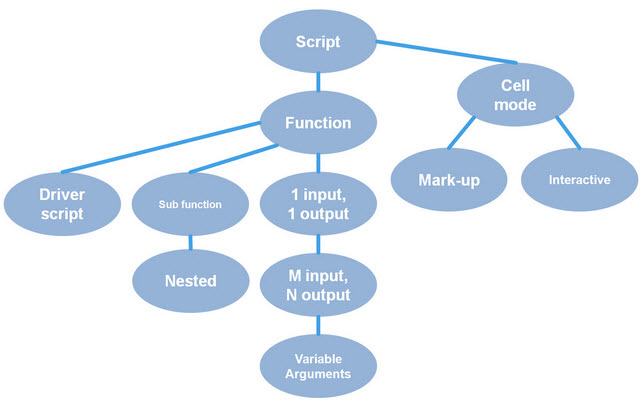Managing code in MATLAB: Functions

This is the first series of MATLAB video tutorials that are an attempt to systematically make videos for a “technology trees” for using MATLAB. This first tree covers the various kinds of MATLAB files you might write. The tree shows you the order you should watch them because some rely on skills you need to learn from earlier videos, but some do not.This video will make a simple function, then the next video will make a different function of one input and one output that we will modify through much of the rest of the tree.

|